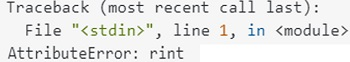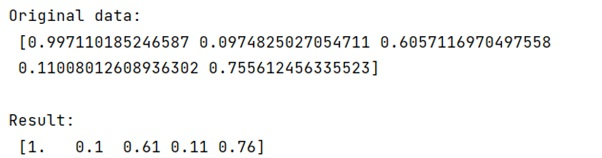# 'AttributeError: rint' when using numpy.round()

Learn, how can we fix 'AttributeError: rint' when using numpy.round() in Python?
Submitted by Pranit Sharma, on March 20, 2023

## Fixing - 'AttributeError: rint' when using numpy.round()

Suppose that we are given a numpy array and we need to round the numbers in the array up to two decimal places. We can use numpy.round() or numpy.around() but sometimes we can face the following error:This happens because we try to round numpy arrays that are objects. Rather than rounding the numpy objects, we should change them in floats using astype to round the values successfully.

Let us understand with the help of an example,

## Python code to fix 'AttributeError: rint' error, when using numpy.round()

```# Import numpy
import numpy as np

# Creating a numpy array
arr = np.random.rand(5).astype(np.object_)

# Display original data
print("Original data:\n",arr,"\n")

# Rounding numpy array
res = np.around(arr.astype(np.double),2)

# Display result
print("Result:\n",res,"\n")
```

### Output